Tutorial math and reading software for elementary and secondary arithmetic, basic math, algebra, geometry, precalculus plus GED, ABE, and CLEP preparation for elementary school, high school, college, adult education, and homeschool students.

# Chapter 3:Linear Equations in TWO Variables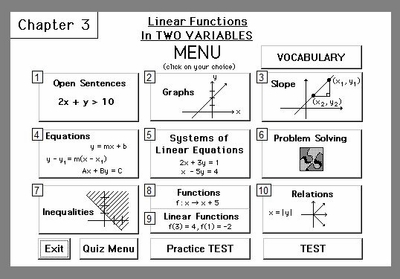Item# AlgCh3
Regular price: \$49.00
Limited Time Offer: \$24.50
Media type::

## Product Description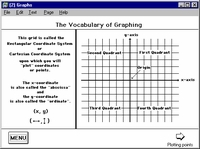[Click on the images to enlarge for better viewing.]

Students will solve linear equations and open sentences in two variables, learn to plot and graph linear equations and linear inequalities. Solving equations and graphing linear equations is a key algebra concept and the foundation of further math study. Chapter 3 continues with an in-depth study of the slope of a line and equations of a line: slope-intercept, point-slope, standard form. Study of graphing linear equations and solving equations continues with graphing systems of linear equations to find the solution and solving a system of linear equations by linear combination. Word problem applications are practiced and understanding functions and relations is studied in detail. A test of understanding concludes this program. The linear equations test is offered as a study guide with hints and solutions as well as a test for scoring and recording progress. (Functionality includes automatic scoring and recording of assessments as well as "bookmarking" for the student to return to the last screen they worked on in their previous session.)

## Accessories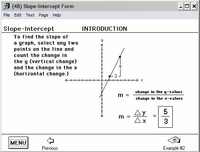Lesson introductionsTheories are explained with examples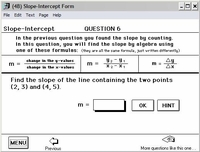Lessons offer practice questionswith hints and solutions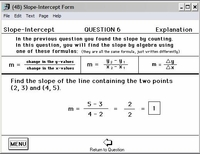Every question has detailed solutions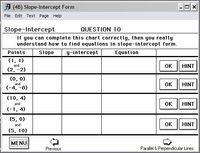Questions are VariedKeeps learning interesting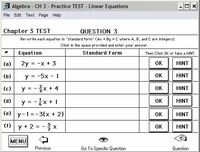Practice Testswith hints and complete solutions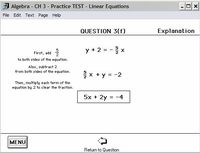Complete solutions - available upon demand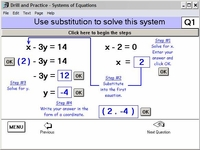Systems of Equations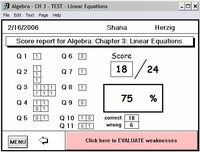After the practice test, take the "real" test for a score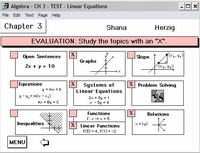Weaknesses are evaluated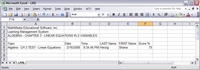Scores are stored